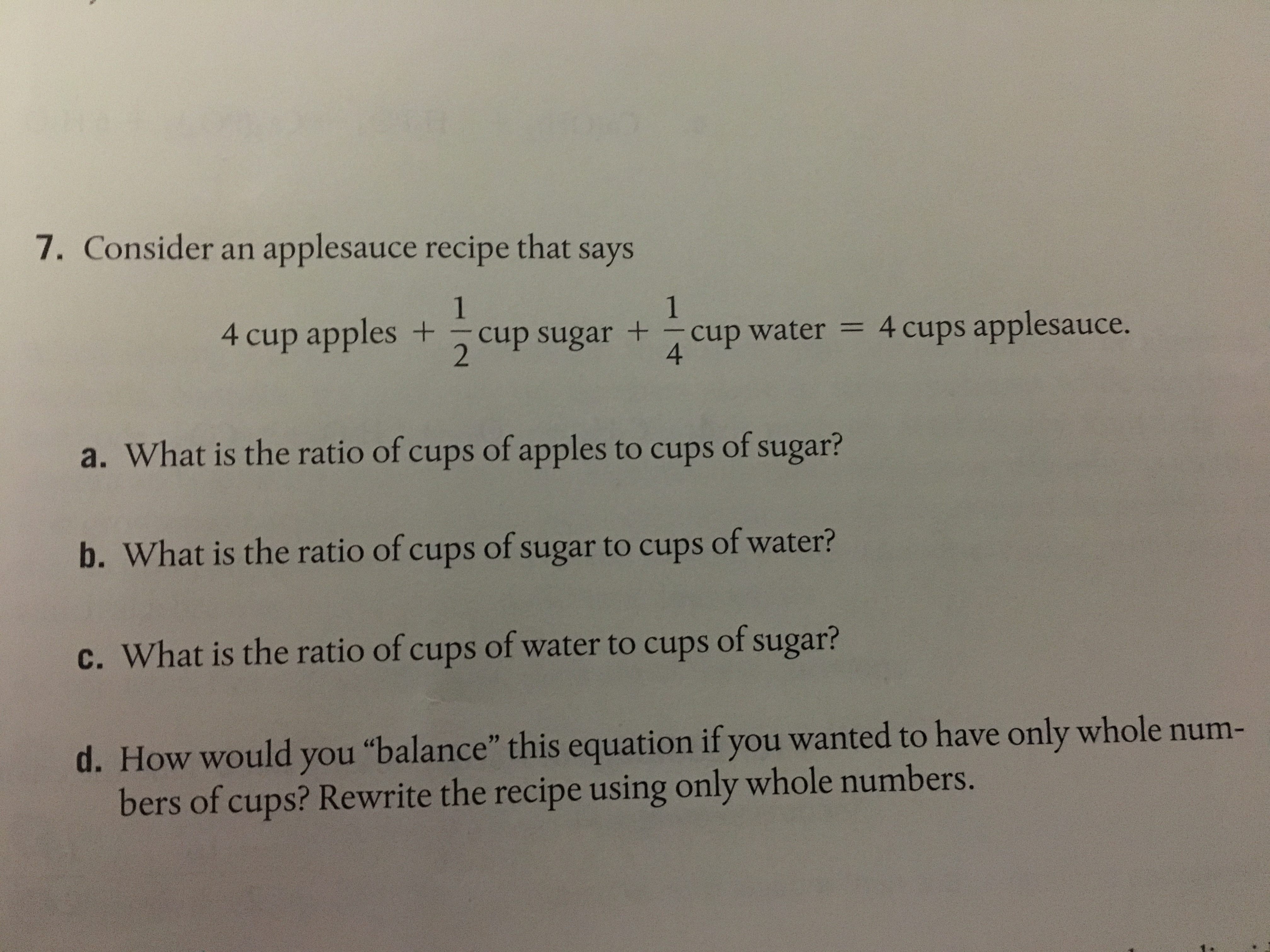7. Consider an applesauce recipe that says4 cup apples +-cup sugar +-cup water = 4 cups applesauce.42a. What is the ratio of cups of apples to cups of sugar?b. What is the ratio of cups of sugar to cups of water?c. What is the ratio of cups of water to cups of sugar?d. How would you "balance' this equation ifyou wanted to have only whole num-bers of cups? Rewrite the recipe using only whole nu

Questionhelp_outlineImage Transcriptionclose7. Consider an applesauce recipe that says 4 cup apples +-cup sugar +-cup water = 4 cups applesauce. 4 2 a. What is the ratio of cups of apples to cups of sugar? b. What is the ratio of cups of sugar to cups of water? c. What is the ratio of cups of water to cups of sugar? d. How would you "balance' this equation ifyou wanted to have only whole num- bers of cups? Rewrite the recipe using only whole nu fullscreen
Step 1

Hi. Since this question has multiple parts and we can answer only first 3 parts, so here is the solution.

For the first part it says ratio of cups of apple to cups of sugar

Step 2

The ratio of cups of apple to cups of sugar is 8 : 1

Step 3

For the second part, it is required to calculate t...

Want to see the full answer?

See Solution

Want to see this answer and more?

Our solutions are written by experts, many with advanced degrees, and available 24/7

See Solution
Tagged in

Other